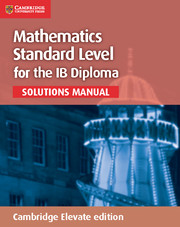Cart
× This is a Sterling guide price. You will be invoiced in the appropriate currency for your country.
• ISBN:9781107579408
• Format:Cambridge Elevate
• Subject(s):Mathematics
• Author(s):Paul Fannon, Vesna Kadelburg, Ben Woolley, Stephen Ward
• Available from: May 2016

This is a series of fully worked solutions manuals for Mathematics Standard Level for the IB Diploma and Mathematics Higher Level for the IB Diploma.

× This is a Sterling guide price. You will be invoiced in the appropriate currency for your country.

Send a Query

formRow
×
• DESCRIPTION

This Cambridge Elevate Solutions Manual for Mathematics for the IB Diploma Higher Level contains approximately 1250 fully worked solutions to the colour-coded examination-style questions contained in the coursebook. The solutions detail one method of solving the problem, with comments to give additional explanations where required.

• Contents
• CONTENTS
• 1. Counting principles
• 2. Exponents and logarithms
• 3. Polynomials
• 4. Algebraic solutions
• 5. The theory of functions
• 6. Transformations of graphs
• 7. Sequences and series
• 8. Binomial expansion
• 9. Circular measure and trigonometric functions
• 10. Trigonometric equations and identities
• 11. Geometry of triangles and circles
• 12. Further trigonometry
• 13. Vectors
• 14. Lines and planes in space
• 15. Complex numbers
• 16. Basic differentiation and its applications
• 17. Basic integration and its applications
• 18. Further differentiation methods
• 19. Further integration methods
• 20. Further applications of calculus
• 21. Summarising data
• 22. Probability
• 23. Discrete probability distributions
• 24. Continuous distributions
• 25. Mathematical induction
• 26. Questions crossing chapters£17.95 (+VAT) Mathematics for the IB Diploma Standard Level Solutions Manual View product

Not already registered? Create an account now. ×

Other Cambridge websitesNeed a local sales consultant?